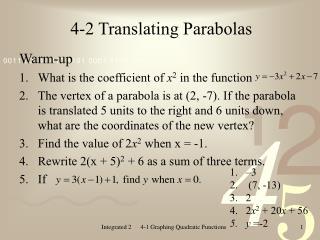DownloadDownload Presentation4-2 Translating Parabolas

# 4-2 Translating Parabolas

Télécharger la présentation## 4-2 Translating Parabolas

- - - - - - - - - - - - - - - - - - - - - - - - - - - E N D - - - - - - - - - - - - - - - - - - - - - - - - - - -
##### Presentation Transcript

1. 4-2 Translating Parabolas Warm-up • What is the coefficient of x2 in the function • The vertex of a parabola is at (2, -7). If the parabola is translated 5 units to the right and 6 units down, what are the coordinates of the new vertex? • Find the value of 2x2 when x = -1. • Rewrite 2(x + 5)2 + 6 as a sum of three terms. • If • –3 • (7, -13) • 2 • 2x2 + 20x + 56 • y =-2 Integrated 2 4-1 Graphing Quadratic Functions

2. 4-1 Graphing Quadratic Functions Today we will: • Explore translations of parabolas. Tomorrow we will: • Solve quadratic equations by graphing and undoing. Integrated 2 4-1 Graphing Quadratic Functions

3. Width of Parabola • The value of a in the function affects the width of the graph. • The greater the absolute value of a, the narrower the graph. Integrated 2 4-1 Graphing Quadratic Functions

4. Example 1 Tell whether the graph of each function is wider than, narrower than, or the same shape as the graph of y = x2. • Solution • The value of a is –3. Since |-3| > 1, the graph is narrower than the graph of y = x2. • In standard form, this function is . The value of a is –1. Since |-1| = 1, this graph has the same shape as the graph y = x2. • The value of a is 2/3. Since | 2/3 | < 1, this graph is wider than the graph of y = x2. Integrated 2 4-1 Graphing Quadratic Functions

5. Example 2 Arrange these quadratic functions in order from the one with the narrowest graph to the one with the widest graph. Solution Integrated 2 4-1 Graphing Quadratic Functions

6. Translations • The graph of is a translation of the graph . • A translation changes only the position of a graph; • it doesn’t change the size or shape of the graph, or • the direction that the graph opens. Integrated 2 4-1 Graphing Quadratic Functions

7. Translations Subtraction indicates a translation to the right. Addition indicates a translation to the left. Translations can also occur in two directions at the same time. A graph can be translated to the right and up, to the right and down, to the left and up, and to the left and down. Integrated 2 4-1 Graphing Quadratic Functions

8. Solution: • To translate the graph of 3 units to the right, replace x with (x-3). • To translate the graph of down 5 units, subtract 5 from . • The equation of the parabola is Example 3 Give the equation of the parabola that results when the graph of is translated 5 units down and 3 units to the right. Integrated 2 4-1 Graphing Quadratic Functions

9. Solution: • To translate the graph of 7 units to the left replace x with (x+7). • To translate the graph of up 1 unit, add 1 to . Example 4 Give the equation of the parabola that results when the graph of is translated 7 units to the left and 1 unit up. Integrated 2 4-1 Graphing Quadratic Functions

10. Example 5 Tell how to translate the graph of in order to produce the graph of each function. Translate 9 units left Translate 9 units up Translate 2 units right and 1 unit up Translate 12 units right and 3 units down Integrated 2 4-1 Graphing Quadratic Functions

11. Find the coordinates of the vertex of the graph of Solution The graph of is a translation of the graph 6 units left and 8 units up. The vertex of the graph of is 6 units to the left and 8 units up from the vertex of the graph of , which is (0, 0). (0 – 6, 0 + 8) (-6, 8) Example 6 The coordinates of the vertex are (-6, 8) Integrated 2 4-1 Graphing Quadratic Functions